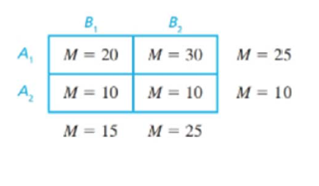Chapter 13.3, Problem 1LCEssentials of Statistics for the B...

8th Edition
Frederick J Gravetter + 1 other
ISBN: 9781133956570

Solutions

Chapter
SectionEssentials of Statistics for the B...

8th Edition
Frederick J Gravetter + 1 other
ISBN: 9781133956570
Textbook Problem

The following matrix shows the results from a two-factor experiment.a. What means are compared to evaluate the main effect for factor A ?b. What means are compared to evaluate the main effect for factor B?c. Does there appear to be an interaction between the two factors? Explain answer.a.

To determine

To find: Which means are compared to evaluate the main effect for factor A.

Explanation

Given info:

The information is representing in the matrix with two-factor experiment.

Calculation:

By observing the matrix,

Thus, the main effect for factor A compares

b.

To determine

To find: Which means are compared to evaluate the main effect for factor B.

c.

To determine

To find: Is there an interaction between the two factor.

Still sussing out bartleby?

Check out a sample textbook solution.

See a sample solution

The Solution to Your Study Problems

Bartleby provides explanations to thousands of textbook problems written by our experts, many with advanced degrees!

Get Started

Simplify each radical expression. 10036

Trigonometry (MindTap Course List)

The slope of the tangent line to y = x3 at x = 2 is: 18 12 6 0

Study Guide for Stewart's Single Variable Calculus: Early Transcendentals, 8th

True or False: The function f is continuous at (0, 0), where

Study Guide for Stewart's Multivariable Calculus, 8th